Question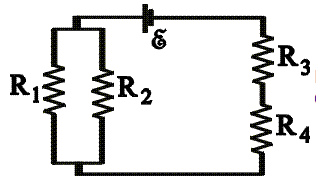Consider the circuit shown below: R1 = 600 ohm R2 = 480 ohm R3 = 240 ohm R4 = 210 ohm ε = 4.5 V a)Calculate the potential difference across R4. b)Calculate the power dissipated in R3.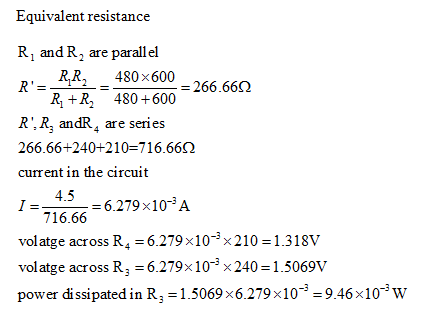#### Earn Coins

Coins can be redeemed for fabulous gifts.

Similar Homework Help Questions
• ### In the circuit below given that R1 = 500 Ohm, R2 = 820 Ohm, R3 =...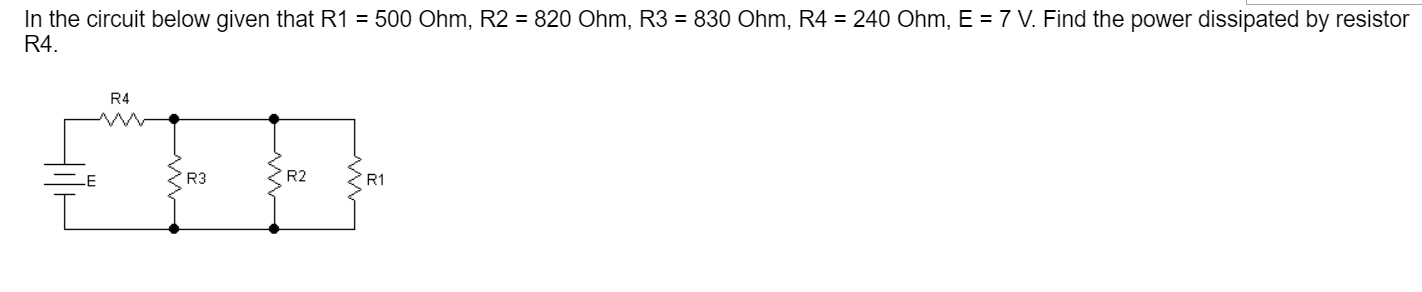In the circuit below given that R1 = 500 Ohm, R2 = 820 Ohm, R3 = 830 Ohm, R4 = 240 Ohm, E = 7 V. Find the power dissipated by resistor R4. R4 E R3 R2 R1

• ### pls help R1 R3 R2 w w V14 V3+ V2+ Consider the circuit shown below, where...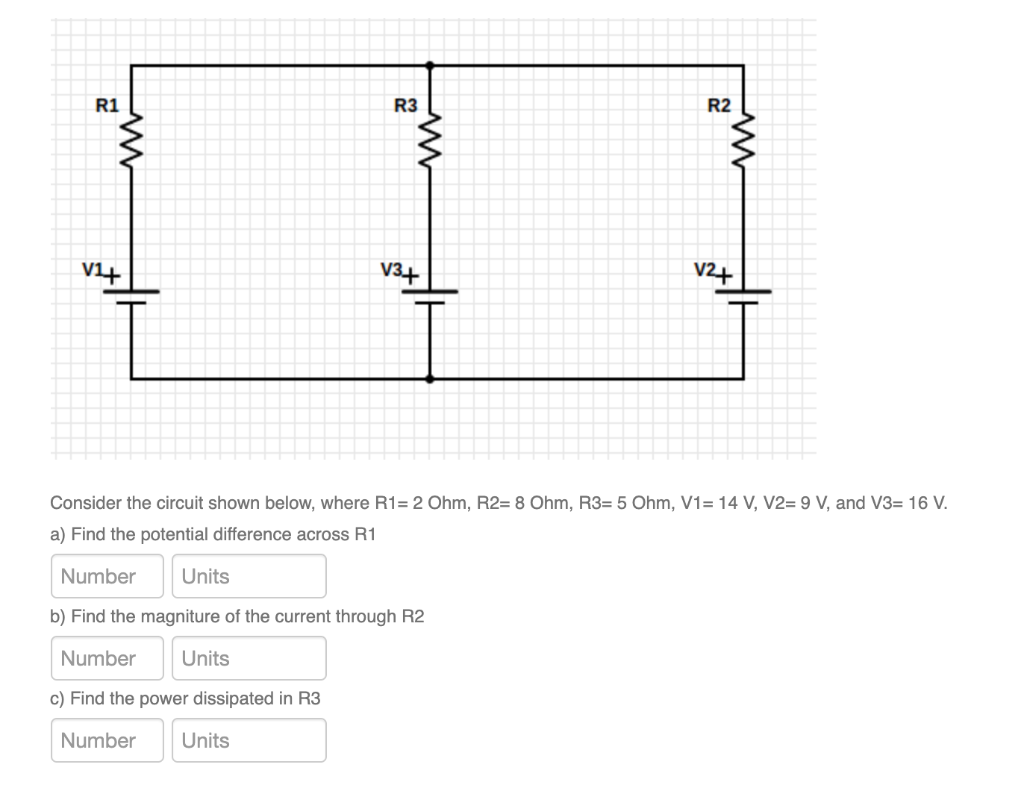pls help R1 R3 R2 w w V14 V3+ V2+ Consider the circuit shown below, where R1= 2 Ohm, R2= 8 Ohm, R3= 5 Ohm, V1= 14 V, V2= 9 V, and V3= 16 V. a) Find the potential difference across R1 Number Units b) Find the magniture of the current through R2 Number Units c) Find the power dissipated in R3 Number Units

• ### Use the circuit shown to fill in the table below. (R1=R2=300 ohm, R3=R4 = 600 ohm)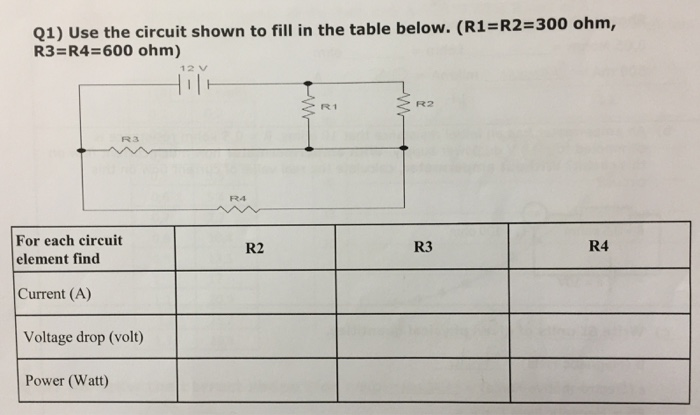Use the circuit shown to fill in the table below. (R1=R2=300 ohm, R3=R4 = 600 ohm)

• ### Ras SR. Consider the circuit, as shown in the figure above. R1 = 12.0 ohm, R2...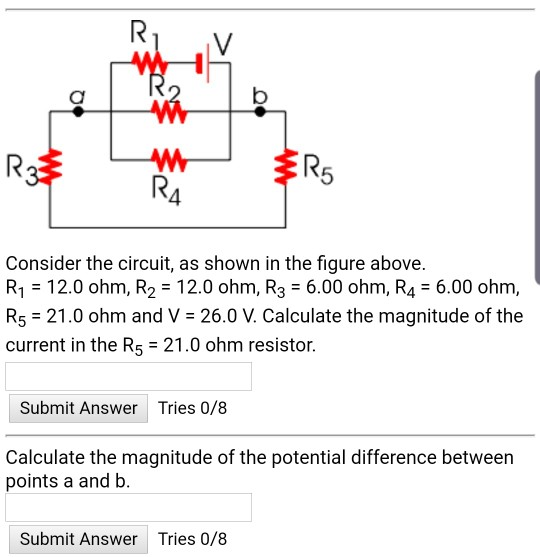Ras SR. Consider the circuit, as shown in the figure above. R1 = 12.0 ohm, R2 = 12.0 ohm, R3 = 6.00 ohm, R4 = 6.00 ohm, R5 = 21.0 ohm and V = 26.0 V. Calculate the magnitude of the current in the R5 = 21.0 ohm resistor. Submit Answer Tries 0/8 Calculate the magnitude of the potential difference between points a and b. Submit Answer Tries 0/8

• ### R5 4 Consider the circuit, as shown in the figure above. R1 = 10.0 ohm, R2...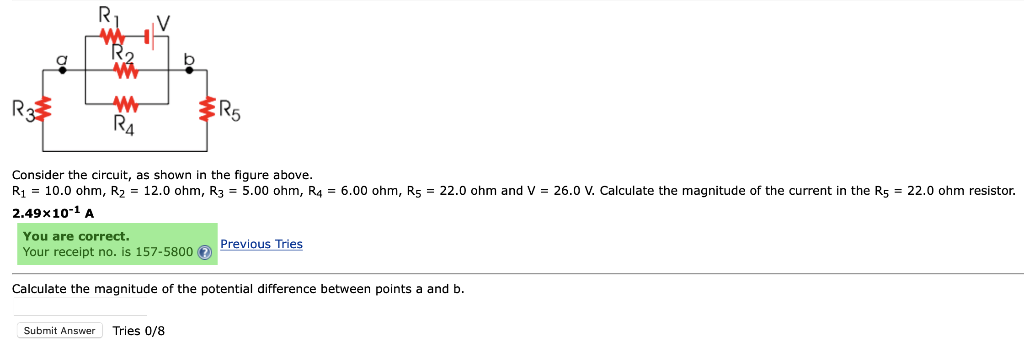R5 4 Consider the circuit, as shown in the figure above. R1 = 10.0 ohm, R2 = 12.0 ohm, R3 = 5.00 ohm, R4 = 6.00 ohm, R5 = 22.0 ohm and V = 26.0 V. Calculate the magnitude of the current in the R5 = 22.0 ohm resistor. 2.49x101 A You are correct Your receipt no. is 157-5800 Previous Tries Calculate the magnitude of the potential difference between points a and b Submit Answer Tries 0/8

• ### R2 R1 R3 R4 E In the following circuit, R1=0.8 4 Ohm, R2-2' 4 Ohm, R3-3'...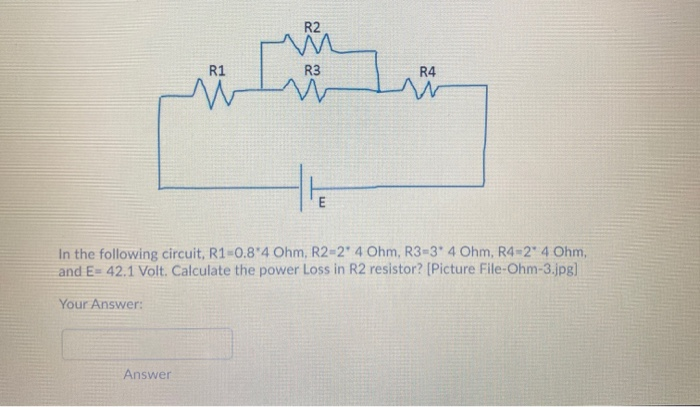R2 R1 R3 R4 E In the following circuit, R1=0.8 4 Ohm, R2-2' 4 Ohm, R3-3' 4 Ohm, R4-2° 4 Ohm, and E= 42.1 Volt. Calculate the power Loss in R2 resistor? [Picture File-Ohm-3.jpg] Your Answer: Answer

• ### Consider the electric circuits below: R1 = 1.00 2: R2 = 2.00 2; R3 =3.002; R4...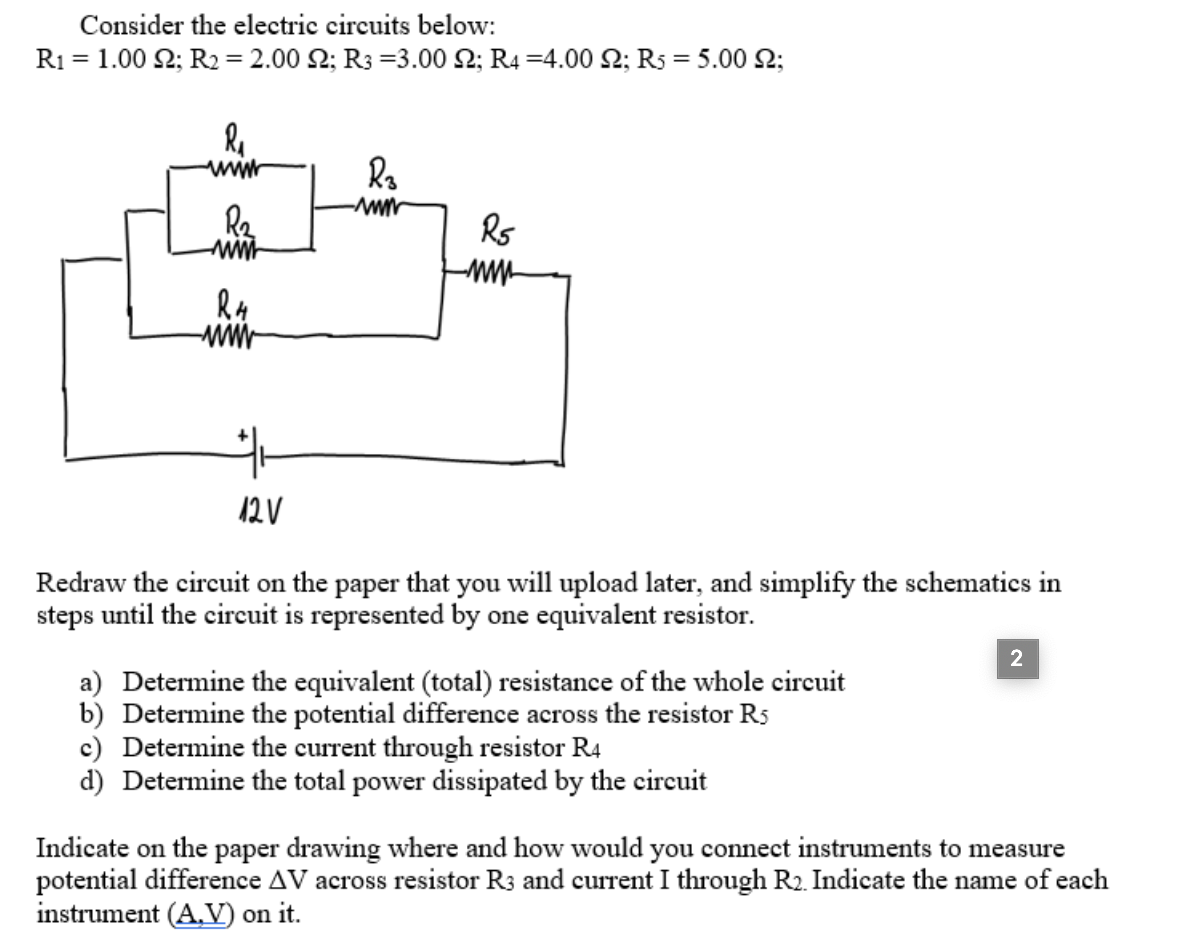Consider the electric circuits below: R1 = 1.00 2: R2 = 2.00 2; R3 =3.002; R4 =4.00 2; Rs = 5.002; R Ro -A R2 Wit R5 RA ww +* 12 V Redraw the circuit on the paper that you will upload later, and simplify the schematics in steps until the circuit is represented by one equivalent resistor. 2 a) Determine the equivalent (total) resistance of the whole circuit b) Determine the potential difference across the resistor Rs c) Determine...

• ### In the circuit shown, R1 = 7 ohm, R2 = 11 ohm, R3 = 9ohm, V1...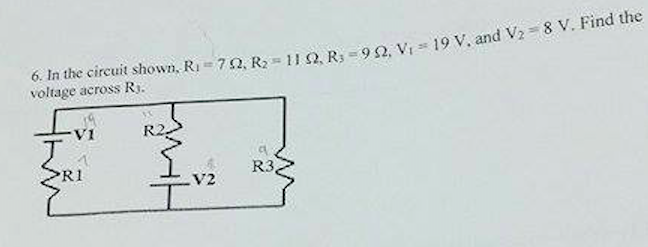In the circuit shown, R1 = 7 ohm, R2 = 11 ohm, R3 = 9ohm, V1 = 19 V, and V2 = 8V. Find the voltage across R3.